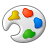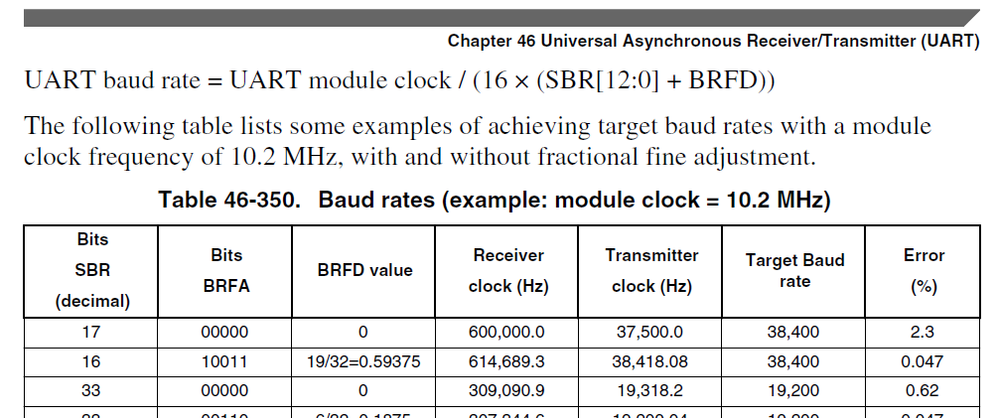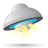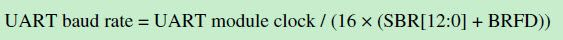## UART (BDH, BDL) of a MK10

cancel
Showing results for
Search instead for
Did you mean:
SOLVED

## UART (BDH, BDL) of a MK10

Jump to solution
946 ViewsContributor III

Hello,
I have to set the registers of the UART (BDH, BDL) of a MK10 to receive a UP501 GPS module.
The GPS baud rate is 9600 (I think the UART baud rate in the formula below).
the UART module clock is 96 MHz (UART module clock in the formula below).

Using the datasheet formula is shown below:I have a much larger value of the size of the registers that I have at our disposal.
How does it work?
In a program I've found is handled as follows.

// compute baud-rate generation values

uint32_t tmp = 2 * *uart_clocks[dev] / baud_rate;  // this equals 32 * (SBR + BRFD)

uart->BDH = tmp >> 13;

uart->BDL = (tmp >> 5) & 0xFF;

I think, that the shift operation is performed because the data is too big but as I understand how shiftare? And then the value does not change?

Thanks for any help

Labels (1)
• ### Kinetis M Series MCUs

1 Solution
234 ViewsNXP TechSupport

Hi

You could find UART baud rate calculate forum is :The code:

// compute baud-rate generation values

uint32_t tmp = 2 * *uart_clocks[dev] / baud_rate;  // this equals 32 * (SBR + BRFD)

The tmp now is 32 * (SBR + BRFD)

The code :

uart->BDH = tmp >> 13;

is actually do two steps calculate:

1> tmp >> 5 (tmp divide by 32), will get (SBR + BRFD) value;

2> (tmp >> 5) >>8 to get SBR[13:9] bit value to UART0_BDH register;

The code :

uart->BDL = (tmp >> 5) & 0xFF;      //make the same calculate.

Wish it helps.

Have a great day,
Ma Hui
-----------------------------------------------------------------------------------------------------------------------
Note: If this post answers your question, please click the Correct Answer button. Thank you!
-----------------------------------------------------------------------------------------------------------------------

3 Replies
234 ViewsNXP TechSupport

Hi

Please refer below code about the UART baud rate calculate:

/********************************************************************/

/*

* Initialize the UART for 8N1 operation, interrupts disabled, and

* no hardware flow-control

*

* NOTE: Since the UARTs are pinned out in multiple locations on most

*      Kinetis devices, this driver does not enable UART pin functions.

*      The desired pins should be enabled before calling this init function.

*

* Parameters:

*  uartch      UART channel to initialize

*  sysclk      UART module Clock in kHz(used to calculate baud)

*  baud        UART baud rate

*/

void uart_init (UART_MemMapPtr uartch, int sysclk, int baud)

{

register uint16 sbr, brfa;

uint8 temp;

/* Enable the clock to the selected UART */

if(uartch == UART0_BASE_PTR)

SIM_SCGC4 |= SIM_SCGC4_UART0_MASK;

else

if (uartch == UART1_BASE_PTR)

SIM_SCGC4 |= SIM_SCGC4_UART1_MASK;

else

if (uartch == UART2_BASE_PTR)

SIM_SCGC4 |= SIM_SCGC4_UART2_MASK;

else

if(uartch == UART3_BASE_PTR)

SIM_SCGC4 |= SIM_SCGC4_UART3_MASK;

else

if(uartch == UART4_BASE_PTR)

SIM_SCGC1 |= SIM_SCGC1_UART4_MASK;

else

SIM_SCGC1 |= SIM_SCGC1_UART5_MASK;

/* Make sure that the transmitter and receiver are disabled while we

* change settings.

*/

UART_C2_REG(uartch) &= ~(UART_C2_TE_MASK

| UART_C2_RE_MASK );

/* Configure the UART for 8-bit mode, no parity */

UART_C1_REG(uartch) = 0;    /* We need all default settings, so entire register is cleared */

/* Calculate baud settings */

sbr = (uint16)((sysclk*1000)/(baud * 16));

/* Save off the current value of the UARTx_BDH except for the SBR field */

temp = UART_BDH_REG(uartch) & ~(UART_BDH_SBR(0x1F));

UART_BDH_REG(uartch) = temp |  UART_BDH_SBR(((sbr & 0x1F00) >> 8));

UART_BDL_REG(uartch) = (uint8)(sbr & UART_BDL_SBR_MASK);

/* Determine if a fractional divider is needed to get closer to the baud rate */

brfa = (((sysclk*32000)/(baud * 16)) - (sbr * 32));

/* Save off the current value of the UARTx_C4 register except for the BRFA field */

temp = UART_C4_REG(uartch) & ~(UART_C4_BRFA(0x1F));

UART_C4_REG(uartch) = temp |  UART_C4_BRFA(brfa);

/* Enable receiver and transmitter */

UART_C2_REG(uartch) |= (UART_C2_TE_MASK

| UART_C2_RE_MASK );

}

The UART baud rate register SBR has 12bit setting for integer.

Wish it helps.

Have a great day,
Ma Hui
-----------------------------------------------------------------------------------------------------------------------
Note: If this post answers your question, please click the Correct Answer button. Thank you!
-----------------------------------------------------------------------------------------------------------------------

234 ViewsContributor III

But why in this program must shift??

// compute baud-rate generation values

uint32_t tmp = 2 * *uart_clocks[dev] / baud_rate;  // this equals 32 * (SBR + BRFD)

uart->BDH = tmp >> 13;

uart->BDL = (tmp >> 5) & 0xFF;

235 ViewsNXP TechSupport

Hi

You could find UART baud rate calculate forum is :The code:

// compute baud-rate generation values

uint32_t tmp = 2 * *uart_clocks[dev] / baud_rate;  // this equals 32 * (SBR + BRFD)

The tmp now is 32 * (SBR + BRFD)

The code :

uart->BDH = tmp >> 13;

is actually do two steps calculate:

1> tmp >> 5 (tmp divide by 32), will get (SBR + BRFD) value;

2> (tmp >> 5) >>8 to get SBR[13:9] bit value to UART0_BDH register;

The code :

uart->BDL = (tmp >> 5) & 0xFF;      //make the same calculate.

Wish it helps.

Have a great day,
Ma Hui
-----------------------------------------------------------------------------------------------------------------------
Note: If this post answers your question, please click the Correct Answer button. Thank you!
-----------------------------------------------------------------------------------------------------------------------